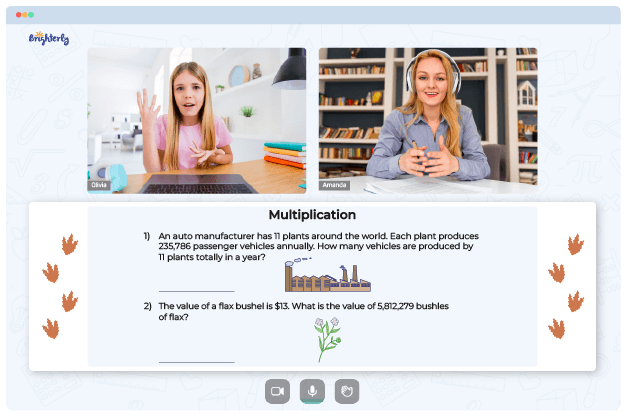# Multiplying Fractions Worksheets

Many teachers agree that the process of teaching fractions is complicated and the topic may seem confusing to students. But kids can’t skip it, especially the fractions multiplication part. Some schools are pushing kids to learn this topic without empowering teachers. Luckily, you can use tools like a multiplying fractions worksheet in PDF format to improve your teaching approach.

## Tips on Using Multiplying Fractions Worksheets

Multiplying fractions worksheets are easy to use because they come with solutions. They show you the process of working through equations. So, even if you don’t understand fractions, you can follow the guide in the worksheets.

Schools have curriculums, and teachers often can devote only limited time to teaching some topics. Sometimes, teachers have to explain some concepts only briefly, reducing the chances of your kids to understand fraction multiplication for example. A multiplying fractions worksheet makes it easy for kids to learn to multiply fractions at a convenient pace.

Math for Kids

Is Your Child Struggling With Math?
1:1 Online Math TutoringUsing a worksheet in the studying process, your kids will do better because they have handy reference points. Secondly, children tend to thrive better in a less competitive atmosphere – their homes. Fractions worksheets can help you teach kids the concept without pressuring them to understand it when they aren’t ready.

Combining formal and playful methods to help your kids understand fraction multiplication is an effective method. In addition to fractions worksheets, you can use games. Games are fun because they also give kids an avenue to explore their creativity through competitive challenges.### Fraction multiplied by a fraction

Fraction Multiplication Worksheet### Fraction multiplied by a whole number

Multiply Fractions Worksheet### Multiplying fractions by whole numbers### Multiplying fractions practice

Multiplying Fractions Worksheet

## Brighterly’s Tutors Teach Kids to Multiply with Fraction Worksheets

Fractions are complex to solve, so investing in an online tutor’s services is a good step toward helping your children improve math skills. A tutor may help your child learn fractions even after coming home from school.

Brighterly provides its tutors with resources that fit your children’s educational needs. It is an online platform that accommodates kids of all ages. The teaching strategy allows kids to learn at comfortable speeds because the tutors understand that every child is unique.

The multiplying fractions worksheets that Brighterly tutors use are also handy for parents who want to revise math with their kids. But if you have a busy schedule, you should let the experienced tutors at Brighterly do the teaching while you relax.

### Fractions Worksheets

Need help with Multiplication?• Does your child need extra support with mastering the concept of multiplication?
• Start lessons with an online tutor.

Is your child finding it hard to understand multiplication? An online tutor could be of help.